Author: Oscar Cronquist Article last updated on March 29, 2018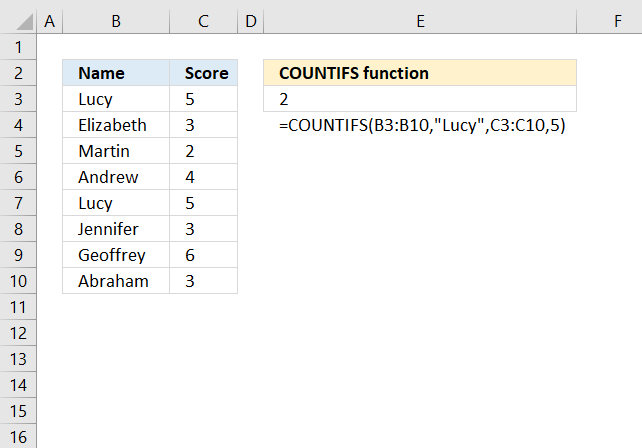The COUNTIFS function calculates the number of cells across multiple ranges that equals all given conditions.

It allows you to use up to 254 arguments or 127 criteria pairs.

Formula in cell E3:

=COUNTIFS(B3:B10,"Lucy",C3:C10,5)

Lucy and 5 are found twice, in row 3 and 7, the function returns 2 in cell E3.

Excel Function Syntax

COUNTIFS(criteria_range1, criteria1, [criteria_range2, criteria2]…)

Arguments

 criteria_range1 Required. The cell range you want to count the cells meeting a condition. criteria1 Required. The condition that you want to count. [criteria_range2] Optional. Additional ranges, up to 127 pairs. [criteria2] Optional. Additional ranges, up to 127 pairs.

To make the function more useful Microsoft made it possible to use wildcards * and question marks ?  in the criteria arguments. ? matches a single character while * matches any sequence of characters.

The really interesting stuff happens when you use the function in an array formula. The number of values in each criteria argument determines how many values the function returns. If one value is used, like in example 1 and 2. A single value is returned.

In example 3 and 4, five values are used in each criteria argument and the COUNTIFS function returns five values. Remember to enter the formulas as an array formula.

Example 1

The following formula counts how many times AA and 10 together exists in cell range B9:B13 and C9:C13.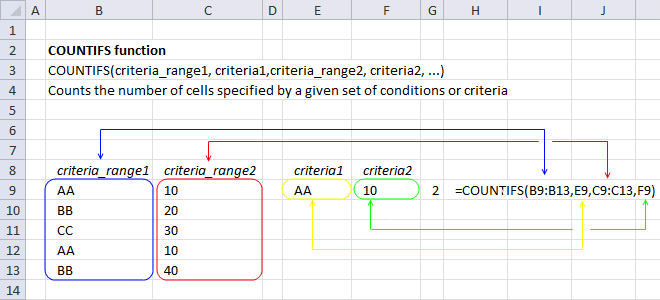COUNTIFS(B9:B13,E9,C9:C13,F9)

becomes

COUNTIFS({"AA";"BB";"CC";"AA";"BB"},"AA",{10;20;30;10;40},10)

and returns 2 in cell G9.

Here is a post where I use this technique: Highlight duplicate rows

Example 2

The formula below counts how many times text string "Han" and dates in february 2013 exists in cell range B9:B13 and C9:C13.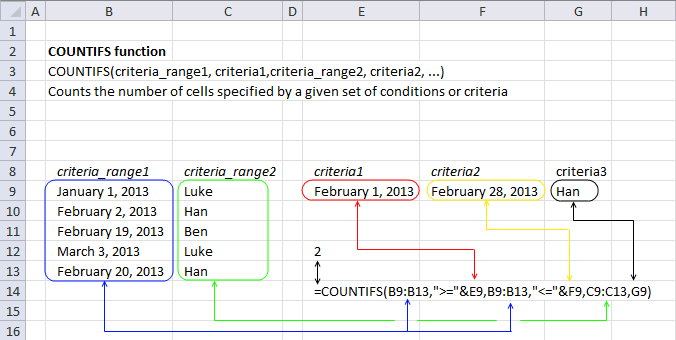=COUNTIFS(B9:B13,">="&E9,B9:B13,"<="&F9,C9:C13,G9)

becomes

=COUNTIFS({41275;41307;41324;41336;41325},">="&41306,{41275;41307;41324;41336;41325},"<="&41333,{"Luke";"Han";"Ben";"Luke";"Han"},"Han")

2 is returned in cell E12.

Here is a post where I use comparison operators: Filter overlapping date ranges

Example 3

The following array formula counts each cell value in each row and returns an array.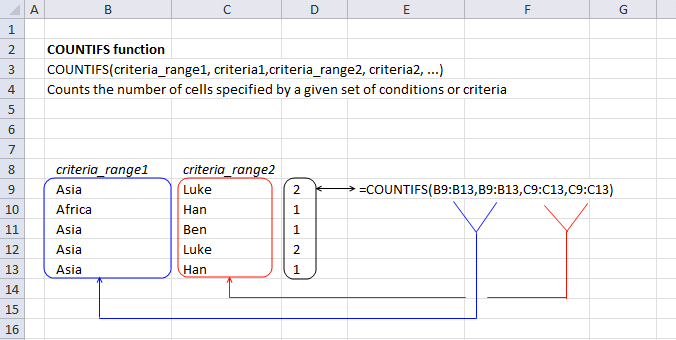=COUNTIFS(B9:B13,B9:B13,C9:C13,C9:C13)

becomes

=COUNTIFS({"Asia"; "Africa"; "Asia"; "Asia"; "Asia"},{"Asia"; "Africa"; "Asia"; "Asia"; "Asia"},{"Luke"; "Han"; "Ben"; "Luke"; "Han"},{"Luke"; "Han"; "Ben"; "Luke"; "Han"})

and returns {2;1;1;2;1} in cell range D9:D13.

Here are two posts where I use this technique:

Example 4

In this example I am using a cell value as a criteria_range and a cell range as criteria. This may seem confusing but it is definitely possible and very useful.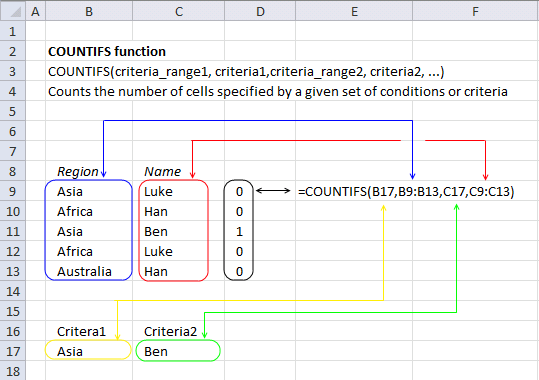=COUNTIFS(B17,B9:B13,C17,C9:C13)

becomes

=COUNTIFS("Asia", {"Asia"; "Africa"; "Asia"; "Africa"; "Australia"}, "Ben", {"Luke"; "Han"; "Ben"; "Luke"; "Han"})

and returns {0; 0; 1; 0; 0} in cell range D9:D13.